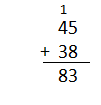# Texas Go Math Grade 2 Lesson 7.1 Answer Key 2-Digit Addition

Refer to our Texas Go Math Grade 2 Answer Key Pdf to score good marks in the exams. Test yourself by practicing the problems from Texas Go Math Grade 2 Lesson 7.1 Answer Key 2-Digit Addition.

## Texas Go Math Grade 2 Lesson 7.1 Answer Key 2-Digit Addition

Explore

Draw quick pictures to model each problem.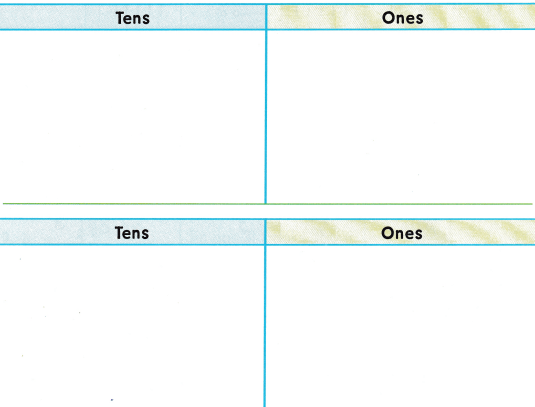FOR THE TEACHER • Read the following problem and have children draw quick pictures to solve. The animal shelter has 35 dogs and 47 cats for adoption. How many pets are at the shelter? Repeat the activity with this problem. After a pet fair, there are only 18 dogs and 21 cats at the shelter. How many pets are at the shelter now?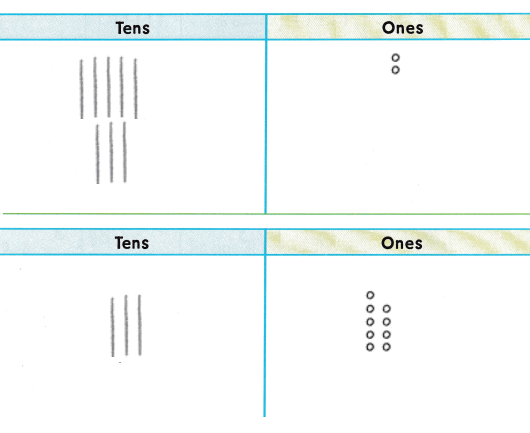Explanation:
Ones and tens are added separately
35 + 47 = 82
18 + 21 = 39

Math Talk
Mathematical Processes

Explain when you need to regroup ones.
When the sum is more than 10 we have to regroup them
to tens and ones.

Model and Draw

Add 59 and 24.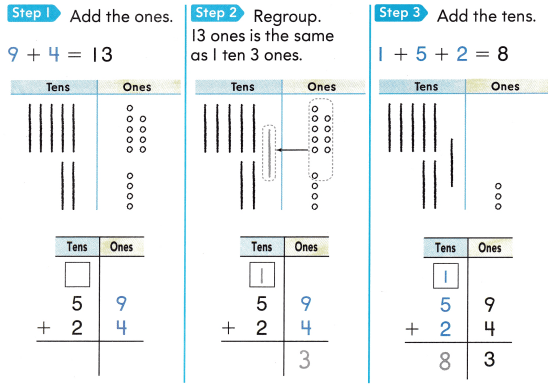59 + 24 = 83
ones are added first then regrouped them to tens and ones
then the carry is added to tens

Share and Show

Regroup if you need to. Write the sum.

Question 1.Explanation:
First we have to add the ones
if the sum is more than ten we have to regroup
to tens and ones

Question 2.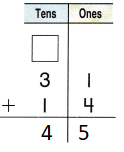Explanation:
First we have to add the ones
if the sum is more than ten we have to regroup
to tens and ones

Question 3.Explanation:
First we have to add the ones
if the sum is more than ten we have to regroup
to tens and ones

Problem Solving

Regroup if you need to. Write the sum.

Question 4.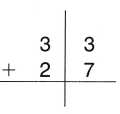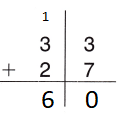Explanation:
First we have to add the ones
if the sum is more than ten we have to regroup
to tens and ones

Question 5.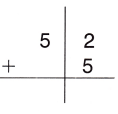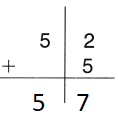Explanation:
First we have to add the ones
if the sum is more than ten we have to regroup
to tens and ones

Question 6.Explanation:
First we have to add the ones
if the sum is more than ten we have to regroup
to tens and ones

Question 7.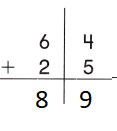Explanation:
First we have to add the ones
if the sum is more than ten we have to regroup
to tens and ones

Question 8.Explanation:
First we have to add the ones
if the sum is more than ten we have to regroup
to tens and ones

Question 9.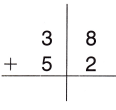Explanation:
First we have to add the ones
if the sum is more than ten we have to regroup
to tens and ones

Solve. Write or draw to explain.

Question 10.
H.O.T. Multi-Step Jin has 31 books about cats and 19 books about dogs. He gives 5 books to his sister. How many books does Jin have now?_________ books
50 books
Explanation:
Jin has 31 books about cats
and 19 books about dogs.
He gives 5 books to his sister.
31 + 19 – 5 = 50
50 books that Jin have now

Question 11.
Abby used a different way to add. Describe Abby’s way of adding 2-digit numbers.The way Abby used is the wrong addition
we have to regroup the tens
Explanation:
35 + 48 = 118Choose the correct answer.

Question 12.
Connect A shark grew 18 new teeth in one week. It grew 17 more new teeth the next week. How many new teeth did the shark grow in two weeks?
(A) 35
(B) 28
(C) 37
Explanation:
A shark grew 18 new teeth in one week.
It grew 17 more new teeth the next week.
18 + 17 = 35
35 new teeth that the shark grow in two weeks

Question 13.
A shark swam 48 miles on Monday. On Tuesday, the shark swam another 48 miles. How many miles did the shark swim in all on these two days?
(A) 18
(B) 96
(C) 86
Explanation:
A shark swam 48 miles on Monday.
On Tuesday, the shark swam another 48 miles.
48 + 48 = 96
96 miles that the shark swim in all on these two days

Question 14.
Reasoning Anna has 31 animal cards. Roy has 49 animal cards. How many animal cards do the two children have?
(A) 79
(B) 70
(C) 80
Explanation:
Reasoning Anna has 31 animal cards.
Roy has 49 animal cards.
49 + 31 = 80
80 animal cards that the two children have

Question 15.
TEXAS Test Prep Carlos had 68 stamps in his collection. He bought 14 more stamps. How many stamps does he have now?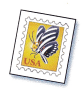(A) 74
(B) 82
(C) 56
Explanation:
Carlos had 68 stamps in his collection.
He bought 14 more stamps.
68 + 14 = 82
82 stamps that he have now.

TAKE HOME ACTIVITY • Ask your child to show you two ways to add 45 and 38.